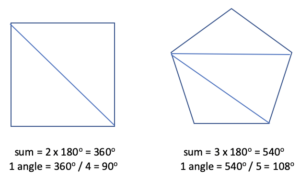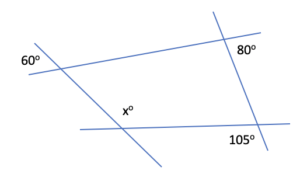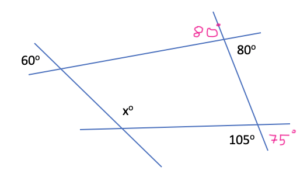# Angles in polygons

### QUESTION #1

What is the sum of the angles in these regular polygons?What is the measure of each angle?

A triangle has 180 degrees.

Let’s see how many triangles can we see in each each shape.Note: A regular polygon has all sides and all angles equal.

### Extend

What is the sum of angles of the regular polygon below? What is the measure of each angle?### QUESTION #2

What is the value of x?HINTS

• opposite angles are equal
• angles that form a straight line have a sum of 180 degrees### QUESTION #3

What is the value of x?